# Complete Symmetric Figures (1)

In this worksheet, students must complete shapes so that they are symmetrical in the given horizontal or vertical line of symmetry.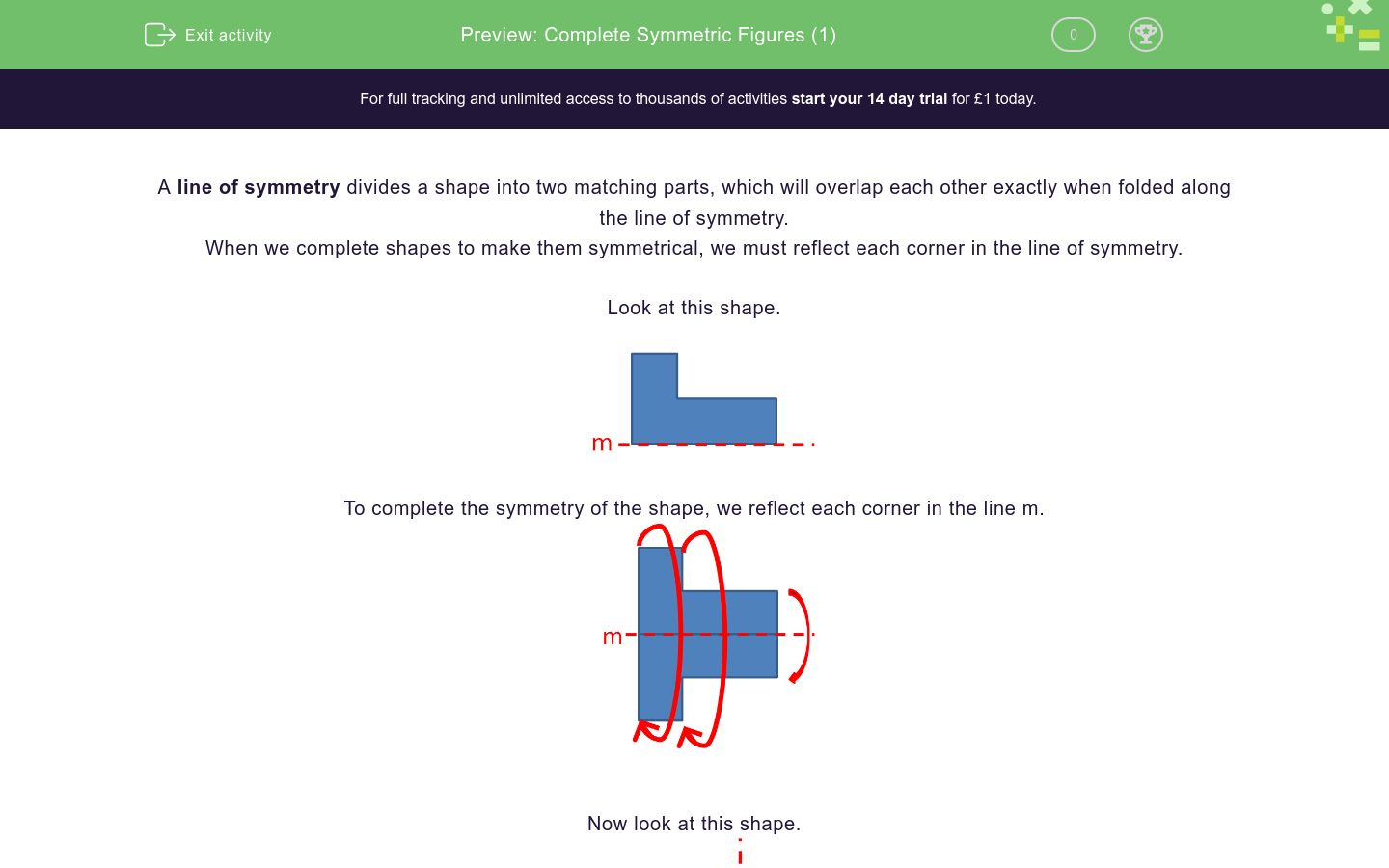Key stage:  KS 2

Curriculum topic:   Geometry: Properties of Shapes

Curriculum subtopic:   Use Symmetry

Difficulty level:### QUESTION 1 of 10

A line of symmetry divides a shape into two matching parts, which will overlap each other exactly when folded along the line of symmetry.

When we complete shapes to make them symmetrical, we must reflect each corner in the line of symmetry.

Look at this shape.To complete the symmetry of the shape, we reflect each corner in the line m.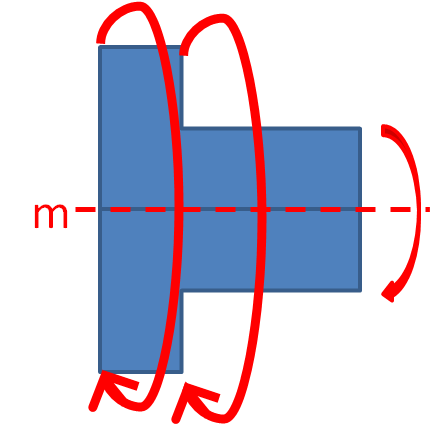Now look at this shape.Notice that there is a gap between the line of symmetry and the shape.

When we reflect the shape, we make sure that there is the same gap on the other side of line m.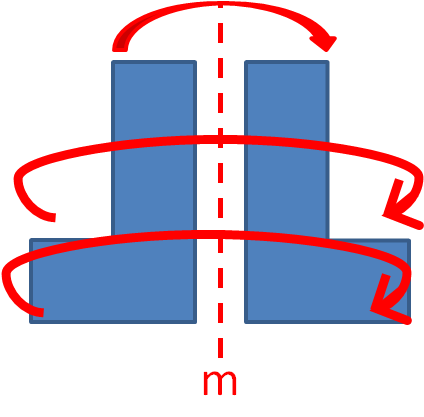Look at this shape.Which diagram shows the completed symmetrical shape?A

B

Look at this shape.Which diagram shows the completed symmetrical shape?A

B

Look at this shape.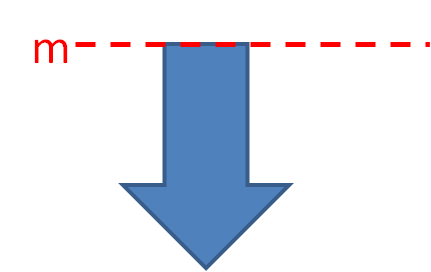Which diagram shows the completed symmetrical shape?A

B

Look at this shape.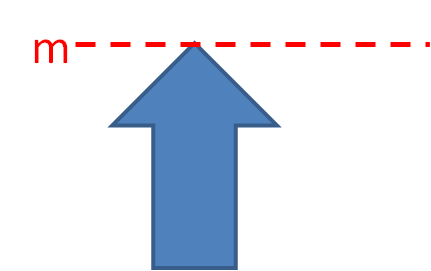Which diagram shows the completed symmetrical shape?A

B

Look at this shape.Which diagram shows the completed symmetrical shape?A

B

Look at this shape.Which diagram shows the completed symmetrical shape?A

B

Look at this shape.Which diagram shows the completed symmetrical shape?A

B

Look at this shape.Which diagram shows the completed symmetrical shape?A

B

Look at this shape.Which diagram shows the completed symmetrical shape?A

B

Look at this shape.Which diagram shows the completed symmetrical shape?A

B

• Question 1

Look at this shape.Which diagram shows the completed symmetrical shape?A
EDDIE SAYS
Shape A is narrow in the middle and wider at the top and bottom.
• Question 2

Look at this shape.Which diagram shows the completed symmetrical shape?A
EDDIE SAYS
Shape A is wider in the middle and narrower at the top and bottom.
• Question 3

Look at this shape.Which diagram shows the completed symmetrical shape?A
EDDIE SAYS
The arrow parts are further away from line m and point in opposite directions when reflected.
• Question 4

Look at this shape.Which diagram shows the completed symmetrical shape?B
EDDIE SAYS
The arrow parts are touching the line m and point in opposite directions when reflected.
• Question 5

Look at this shape.Which diagram shows the completed symmetrical shape?B
EDDIE SAYS
The arrow parts are touching the line m and point in the same direction when reflected vertically.
• Question 6

Look at this shape.Which diagram shows the completed symmetrical shape?B
EDDIE SAYS
The shape does not touch the mirror line m, so there must be a matching space between the shapes.
• Question 7

Look at this shape.Which diagram shows the completed symmetrical shape?B
EDDIE SAYS
The gaps between the shapes and the mirror line m must match.
• Question 8

Look at this shape.Which diagram shows the completed symmetrical shape?A
EDDIE SAYS
The shape formed is like a symmetrical arrowhead.
• Question 9

Look at this shape.Which diagram shows the completed symmetrical shape?A
EDDIE SAYS
The points are at opposite sides and the same distances from the mirror line m.
• Question 10

Look at this shape.Which diagram shows the completed symmetrical shape?B
EDDIE SAYS
The shapes touch at the top and do not touch at the bottom of line m.
---- OR ----

Sign up for a £1 trial so you can track and measure your child's progress on this activity.

### What is EdPlace?

We're your National Curriculum aligned online education content provider helping each child succeed in English, maths and science from year 1 to GCSE. With an EdPlace account you’ll be able to track and measure progress, helping each child achieve their best. We build confidence and attainment by personalising each child’s learning at a level that suits them.

Get started